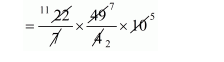# In the middle of a rectangular field measuring 30 m × 20 m,Question:

In the middle of a rectangular field measuring 30 m × 20 m, a well of 7 m diameter and 10 m depth is dug. The earth so removed is evenly spread over the remaining part of the field. Find the height through which the level of the field is raised.

Solution:

Radius of dug $=\frac{7}{2} \mathrm{~m}$

Depth of dug = 10 m

The volume of dug

$=\pi\left(\frac{7}{2}\right)^{2} \times 10$The volume of dug = 385 m3

Let the height through which the level of the field is raised will be x.

But, volume of dug = volume of earth spread over field.

$385=30 \times 20 \times x$

$x=\frac{385}{30 \times 20}$

$x=68.6 \mathrm{~cm}$